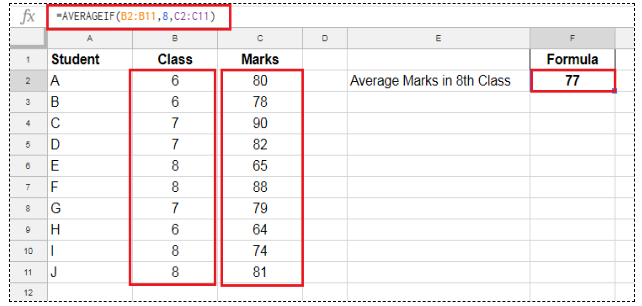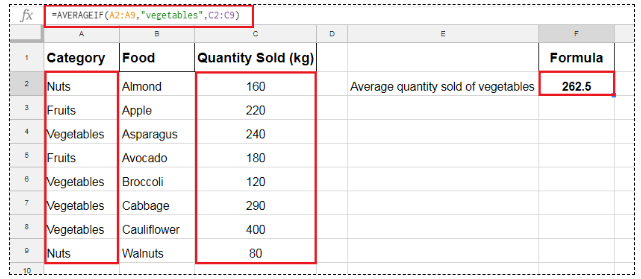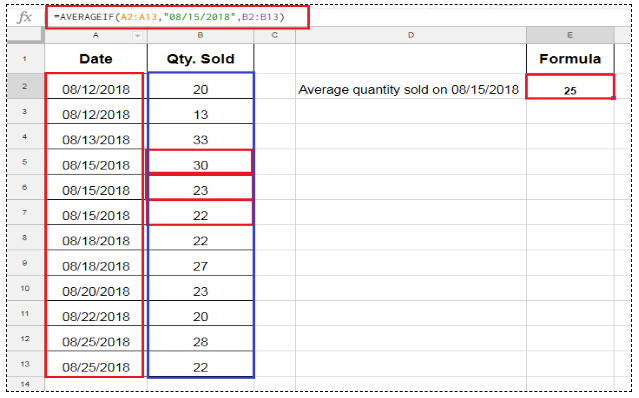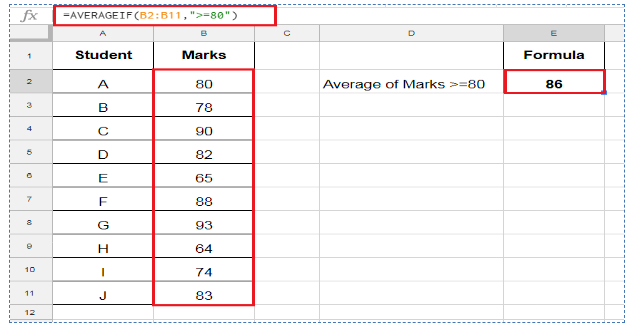Get instant live expert help with Excel or Google Sheets“My Excelchat expert helped me in less than 20 minutes, saving me what would have been 5 hours of work!”

#### Post your problem and you’ll get expert help in seconds.

Your message must be at least 40 characters
Our professional experts are available now. Your privacy is guaranteed.

# How to Use AVERAGEIF in Google Sheets

The Google Sheets AVERAGEIF function returns the average of numbers that meet given criteria in a range. It is basically a combination of AVERAGE and IF functions and acts like an array formula to get the average of resulting array of numbers where a logical condition or criteria is TRUE.

### The AVERAGEIF function in Google Sheets

For example, you have certain numbers, and you want to test a logical condition or criteria on those numbers, and then you need the average of those numbers where a logical condition is TRUE. So you can achieve this by using AVERAGEIF function in Google Sheets.

The syntax of the AVERAGEIF function in Google Sheets is;

`AVERAGEIF(criteria_range, criterion, [average_range])`

Here,

criteria_range – This is a range of values where you need to test logical condition or criteria.

Criterion – This is logical condition or criteria that you want to test on criteria_range. It can consist of a number or text or date or logical expression to test the condition.

average_range- This is a range of numeric values that you want to average. It is an optional argument, and if you do not supply this argument, the AVERAGEIF function takes criteria_range to calculate average.

Here you need to test the various criteria options to calculate the average of numbers that meet these criteria using the Google Sheets AVERAGEIF function.

### AVERAGEIF for Number as criteria

You can use a number as criteria to calculate the average of those numbers that are equal to this criterion number. In this example, you want to calculate average marks of students in class 8th using Google Sheets AVERAGEIF function.

=AVERAGEIF(B2:B11,8,C2:C11)### AVERAGEIF for Text as criteria

If you want to test a text value as criteria on criteria_range then you need to supply the criteria in double quotation marks (“”) in the criterion argument of the Google Sheets AVERAGEIF function. For example, you have data of quantity sold of various foods’ categories, and you need to calculate the average quantity sold of vegetables using AVERAGEIF function in Google Sheets.

`=AVERAGEIF(A2:A9,"vegetables",C2:C9)`### AVERAGEIF for Date as criteria

The average can be calculated based on date as criteria using Google Sheets AVERAGEIF function. The date can be supplied directly or as a cell reference or as a date function like TODAY or DATE function in AVERAGEIF function in Google Sheets.

If you supply the date directly as criteria in the AVERAGEIF function, then you need to enter it in double quotation marks (“”), and if you enter it as cell reference or date function then it will be entered without double quotation marks, such as;

`=AVERAGEIF(A2:A13,"08/15/2018",B2:B13)`

OR

`=AVERAGEIF(A2:A13,Date(2018,8,15),B2:B13)`#### AVERAGEIF for Expression as criteria

Based on criteria expression you can calculate average of numbers in Google Sheets AVERAGEIF function. Like if you want to calculate average of numbers that are Greater Than (>) or Greater Than Equal to (>=) or Less Than (<) or Less Than Equal to (<=) or Not Equal to (<>) to a specified number, then these expressions must be supplied in double quotation marks in criterion argument, such as;

Greater Than “>10”

Greater Than Equal to “>=10”

Less Than “<10”

Less Than Equal to “<=10”

Not Equal to “<>10”

For example, you need to take the average of students’ marks that are Greater Than Equal to 80 then formula would be;

`=AVERAGEIF(B2:B11,">=80")`Still need some help with Excel formatting or have other questions about Excel? Connect with a live Excel expert here for some 1 on 1 help. Your first session is always free.

### Did this post not answer your question? Get a solution from connecting with the expert.Another blog reader asked this question today on Excelchat:
Solution examplesI just need to edit this formula, {=IFERROR(AVERAGEIFS(B6:D6,F6:H6,"FALSE"),AVERAGEIF(B6:D6,"<>0"))}, to return "-" or "0" if there is nothing in column D. I can share the entire worksheet if need be.
Solved by V. A. in 47 minsI contacted you yesterdaya bout how to use the =AVERAGE function, and for real numbers it works fine, but I tried to get the expert to explain to me how to use the =AVERAGE function when the cells have formulas in them that generate '0' value, so the AVERAGE function would ignore these cells in the averaging method, but got no answer. So, I need to know how use the =AVERAGE function so that when there are formulas in the selected cells to average, that EXCEL would ignore those cells like they have real numbers in them, which seems to work fine. Please advise...
Solved by C. J. in 30 minsI need help calculating average time elapsed. It is in dd:hh:mm:ss text format and so I can't calculate using =AVERAGEIF
Solved by O. E. in 11 minsHello good afternoon, I have a problem with excel. I need a formula to compare data between 3 columns and give me the result in a fourth column
Solved by X. B. in 58 minscan you teach me the steps to create a nested if function, and to nest an AND function inside of an IF function?
Solved by A. Q. in 22 mins## Subscribe to Excelchat.coAnother blog reader asked this question today on Excelchat: